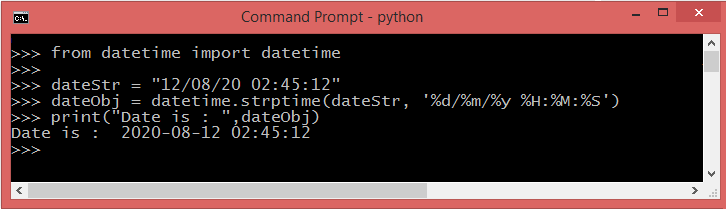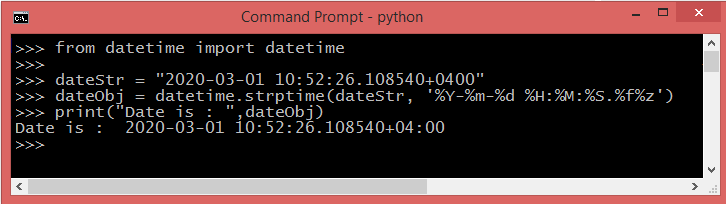You can convert a string to Python date object using the strptime() function (string parse time). It can handle all sorts of formats, with the format determined by a format string you give it:

#### Syntax

``````
strptime(date_string, format)
``````

Return a datetime corresponding to date_string, parsed according to format.The date contains year, month, day, hour, minute, and second.

A reference of all the legal format codes :

Directive Description
%a Weekday as locale's abbreviated name.
%A Weekday as locale’s full name.
%w Weekday as a decimal number, where 0 is Sunday and 6 is Saturday.
%d Day of the month as a zero-padded decimal number.
%b Month as locale’s abbreviated name.
%B Month as locale’s full name.
%m Month as a zero-padded decimal number.
%y Year without century as a zero-padded decimal number.
%Y Year with century as a decimal number.
%H Hour (24-hour clock) as a zero-padded decimal number.
%I Hour (12-hour clock) as a zero-padded decimal number.
%p Locale’s equivalent of either AM or PM.
%M Minute as a zero-padded decimal number.
%S Second as a zero-padded decimal number.
%f Microsecond as a decimal number, zero-padded on the left.
%z UTC offset in the form ±HHMM[SS[.ffffff]] (empty string if the object is naive).
%Z Time zone name (empty string if the object is naive).
%j Day of the year as a zero-padded decimal number.
%U Week number of the year (Sunday as the first day of the week) as a zero padded decimal number. All days in a new year preceding the first Sunday are considered to be in week 0.
%W Week number of the year (Monday as the first day of the week) as a decimal number. All days in a new year preceding the first Monday are considered to be in week 0.
%c Locale’s appropriate date and time representation.
%x Locale’s appropriate date representation.
%X Locale’s appropriate time representation.
%% A literal '%' character.

## Python strptime() ValueError

If string you supplied cannot be parsed according to format, or if it has excess data after parsing, ValueError is raised.

#### example

``````
from datetime import datetime
dateStr = "2020-03-01 10:52:26.108540+04:00"
dateObj = datetime.strptime(dateStr, '%Y-%m-%d %H:%M:%S.%f%z')
print("Date is : ",dateObj)
``````

#### output

``````
ValueError: time data '2018-05-02 11:52:26.108540+02:00' does not match format
%Y-%m-%d %H:%M:%S.%f+%Z'
``````
Here, the issue is the offset +04:00 you need to remove the colon ':' then it will work:©Net-informations.com